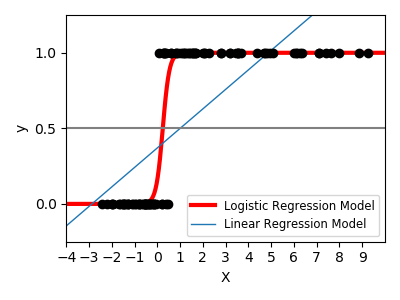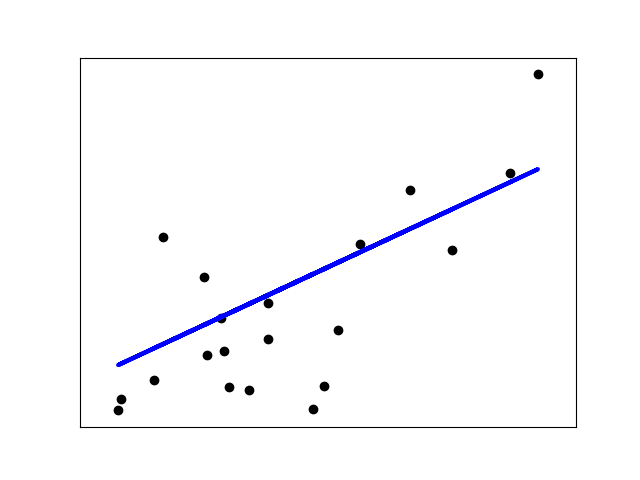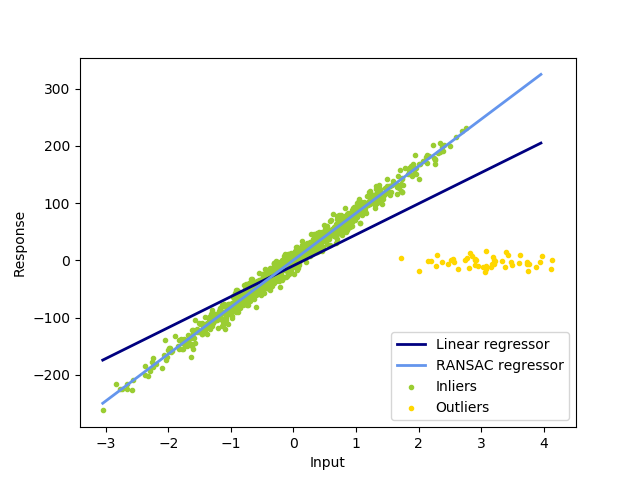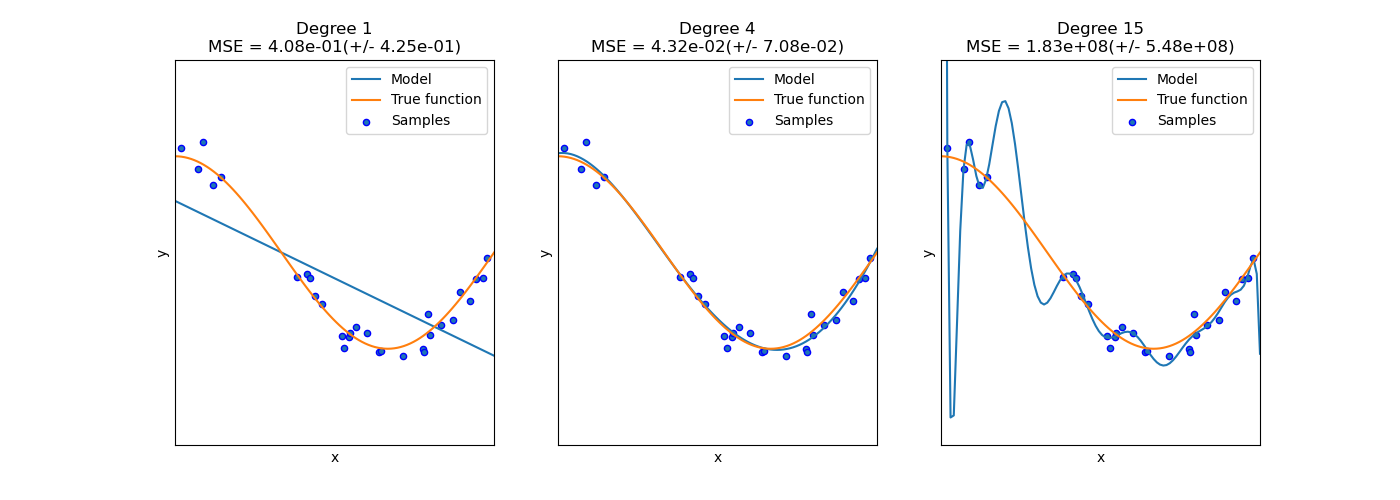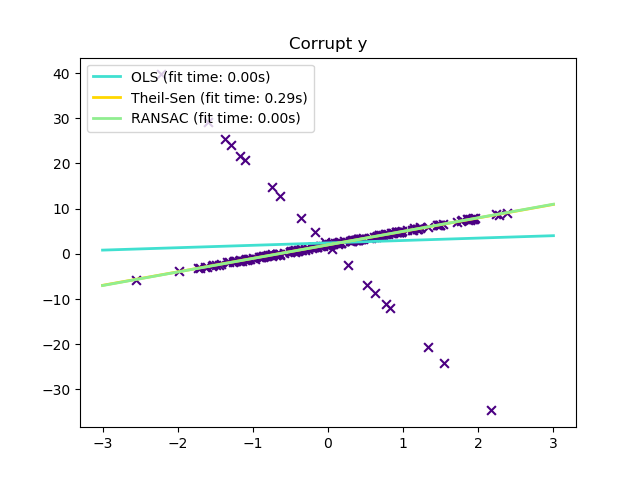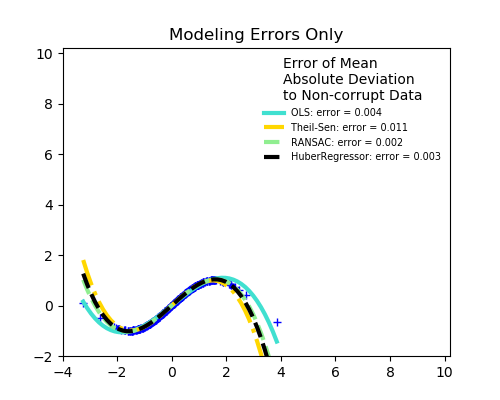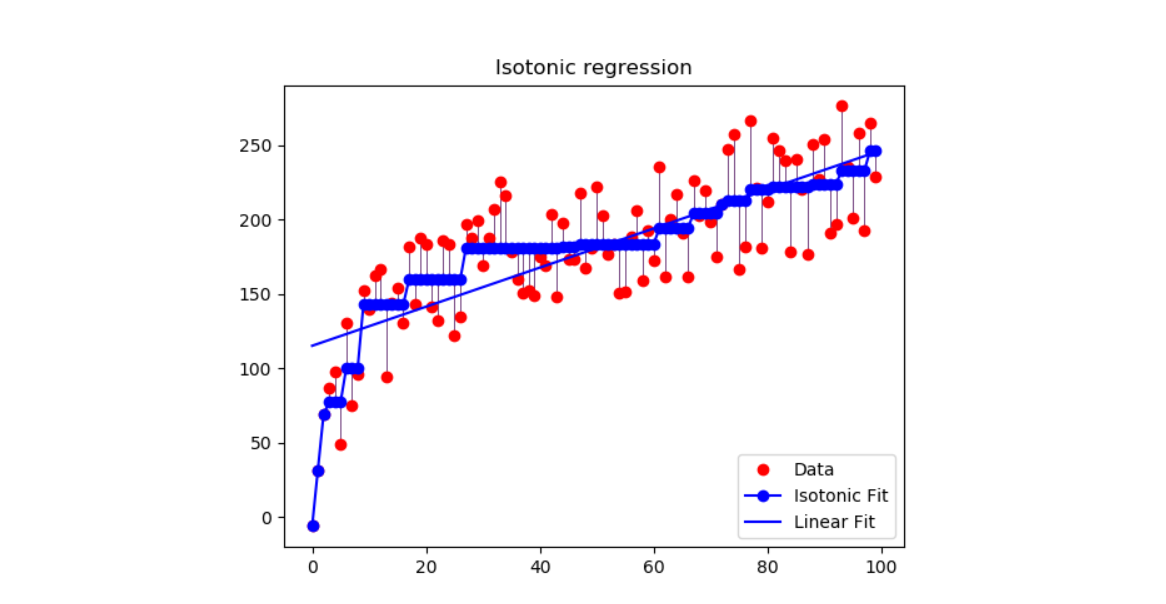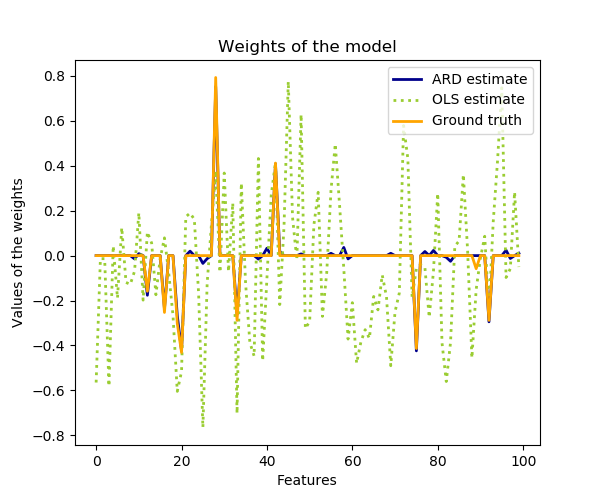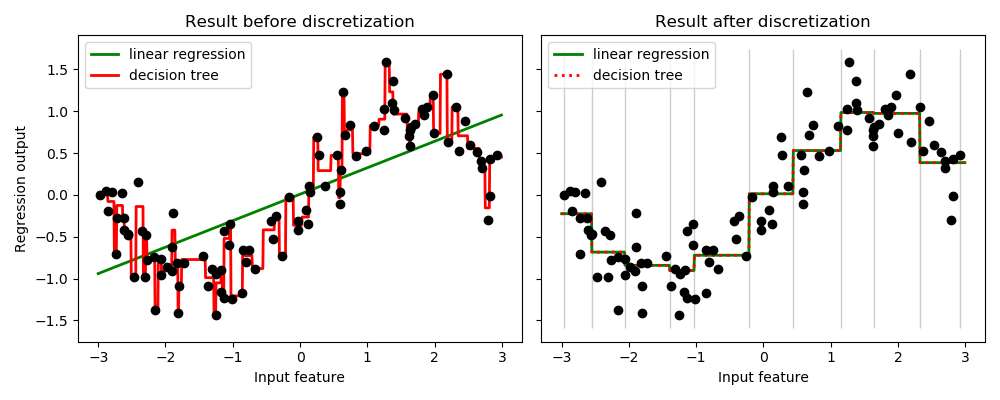# sklearn.linear_model.LinearRegression¶

class sklearn.linear_model.LinearRegression(*, fit_intercept=True, normalize=False, copy_X=True, n_jobs=None)

[源码]

LinearRegression使用系数w =（w1，…，wp）拟合线性模型，以最小化数据集中实际目标值与通过线性逼近预测的目标之间的残差平方和。

fit_intercept bool, default=True

normalize bool, default=False
fit_intercept设置为False 时，将忽略此参数。如果为True，则在回归之前通过减去均值并除以l2-范数来对回归变量X进行归一化。如果你希望标准化，请先使用 sklearn.preprocessing.StandardScaler，然后调用fit 估算器并设置normalize=False
copy_X bool, default=True

n_jobs int, default=None

coef_ array of shape (n_features, ) or (n_targets, n_features)

rank_ int

singular_ array of shape (min(X, y),)
X的奇异值。仅在X是密集矩阵时可用。
intercept_ float or array of shape (n_targets,)

>>> import numpy as np>>> from sklearn.linear_model import LinearRegression>>> X = np.array([[1, 1], [1, 2], [2, 2], [2, 3]])>>> # y = 1 * x_0 + 2 * x_1 + 3>>> y = np.dot(X, np.array([1, 2])) + 3>>> reg = LinearRegression().fit(X, y)>>> reg.score(X, y)1.0>>> reg.coef_array([1., 2.])>>> reg.intercept_3.0000...>>> reg.predict(np.array([[3, 5]]))array([16.])

fit(self, X, y[, sample_weight]) 拟合线性模型。
get_params(self[, deep]) 获取此估计器的参数。
predict(self, X) 使用线性模型进行预测。
score(self, X, y[, sample_weight]) 返回预测的确定系数R ^ 2。
set_params(self, **params) 设置此估计器的参数。
__init__(self, *, fit_intercept=True, normalize=False, copy_X=True, n_jobs=None)

[源码]

fit（self，X，y，sample_weight = None ）

[源码]

X {ndarray, sparse matrix} of shape (n_samples, n_features)

y array-like of shape (n_samples,) or (n_samples, n_targets)

sample_weight array-like of shape (n_samples,), default=None

*0.17版中的新功能：sample_weight参数支持LinearRegression。

self returns an instance of self.

get_params（self，deep = True ）

[源码]

deep bool, default=True

params mapping of string to any

predict(self, X)

[源码]

X array_like or sparse matrix, shape (n_samples, n_features)

C array, shape [n_samples]

score（self，X，y，sample_weight = None ）

[源码]

X array-like of shape (n_samples, n_features)

y array-like of shape (n_samples,) or (n_samples, n_outputs)
X的真实值。
sample_weight array-like of shape (n_samples,), default=None

score float

set_params(self, **params)

[源码]

**params dict

self object

## sklearn.linear_model.LinearRegression使用示例¶